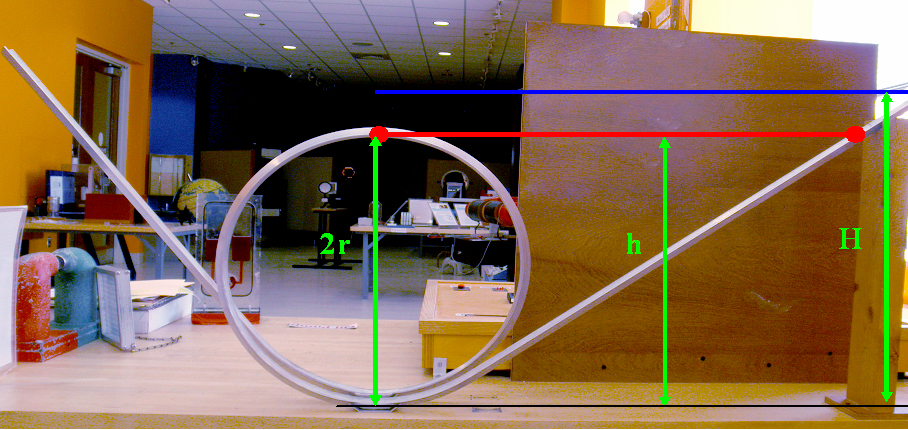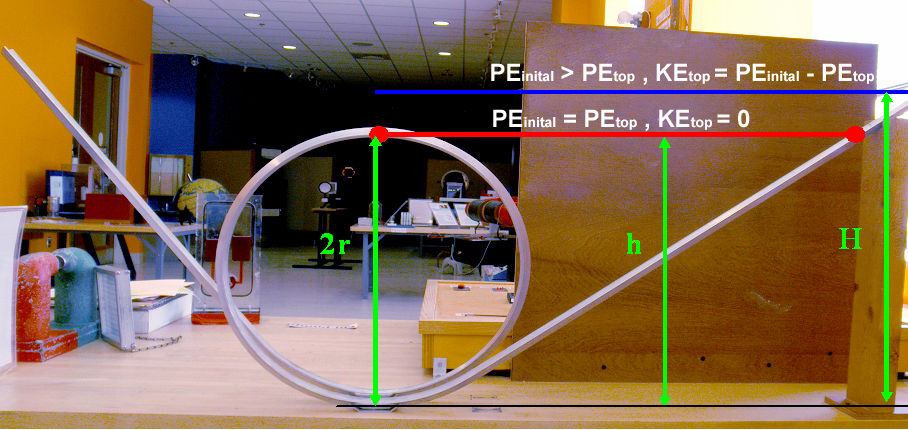# Loop the Loop

The loop the loop is an example of conservation of energy. The three types of energy that we will be considering are: Work, Potential Energy, and Kinetic Energy.

1. Work (W) is the energy given to the object by applying a force over a distance.
2. Potential energy (PE) is the energy the object has due to its position.
3. Kinetic energy (KE)is the energy the object has due to its motion.Conservation of energy states that the total energy of a system remains constant, though it can change forms. There are two lines on the track for the Loop the Loop, one at a height h (which is twice the radius, r, of the loop) and the other at the larger height H.

What to do:

1. Put the ball on the track at a height h above the table (lower line on the track).
2. Release the ball.

What happens when you release the ball at the lower line?
Answer: The ball doesn’t make it around the loop.

What do you THINK will happen when you release the ball at the upper line?

Let’s Try Again And Find Out!

1. Now put the ball on the track at a height H above the table (upper line on the track).
2. Release the ball.

What happens when you release the ball at the upper line?

Answer: The ball makes it around the loop this time!

What happens when you release the ball at other places on the track?

Answer: The ball only makes it around the loop when it is at height H or higher.

What is going on?

You are doing work (W) by raising the ball from the table. Since the ball is at a greater height, it now has an initial potential energy (PEinital) equal to the work you have done. The higher the starting height you give the ball, the greater initial potential energy it has. Whatever PEinital you give to the ball will be the total energy the ball has to travel down the track and around the loop since energy cannot be created or destroyed within the system (conservation of energy)! When you release the ball, it begins to fall down the track acquiring a speed, v. The ball’s potential energy (PE) is being converted into an equal amount of kinetic energy (KE)! At the bottom of the loop, all of the ball’s energy is now kinetic energy.

What happens to the energy after the ball reaches the bottom of the loop?

As the ball starts up the side of the loop, the kinetic energy is being converted back into potential energy, so its kinetic energy and its speed decrease. We know from conservation of energy that the work you have done initially in raising the ball equals the initial potential energy which equals the sum of the kinetic and potential energies at any point on the track.

W= PEinital = PE + KE

When the ball reaches height h at the top of the loop, it needs to still have enough kinetic energy to keep it moving around the loop without falling off.

KEtop= PEinital -PEtop

When we star the ball below height H, the initial potential energy is close to equal the potential energy at the top of the loop, so there is not enough kinetic energy left to keep the ball on the track.The amount of KEtop needed to keep the ball on the track is equal to the work done by the centripetal force to keep it in the loop. This is the force (Fc=mv2/r) needed to keep a mass in a circular motion. The ball will have enough KEtop when it starts above a height H=2.5r, neglecting friction that is. Friction is the energy lost as the ball rolls. This is manly the sound the ball makes as it rolls along the track.

Physics Lecture Demonstration Database